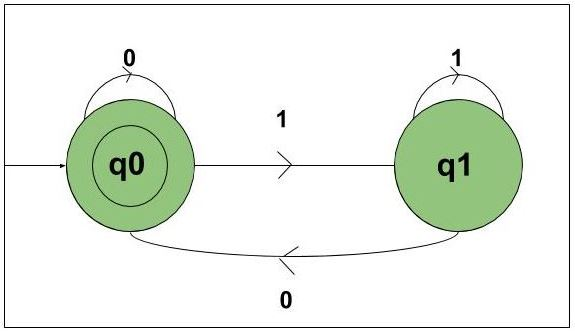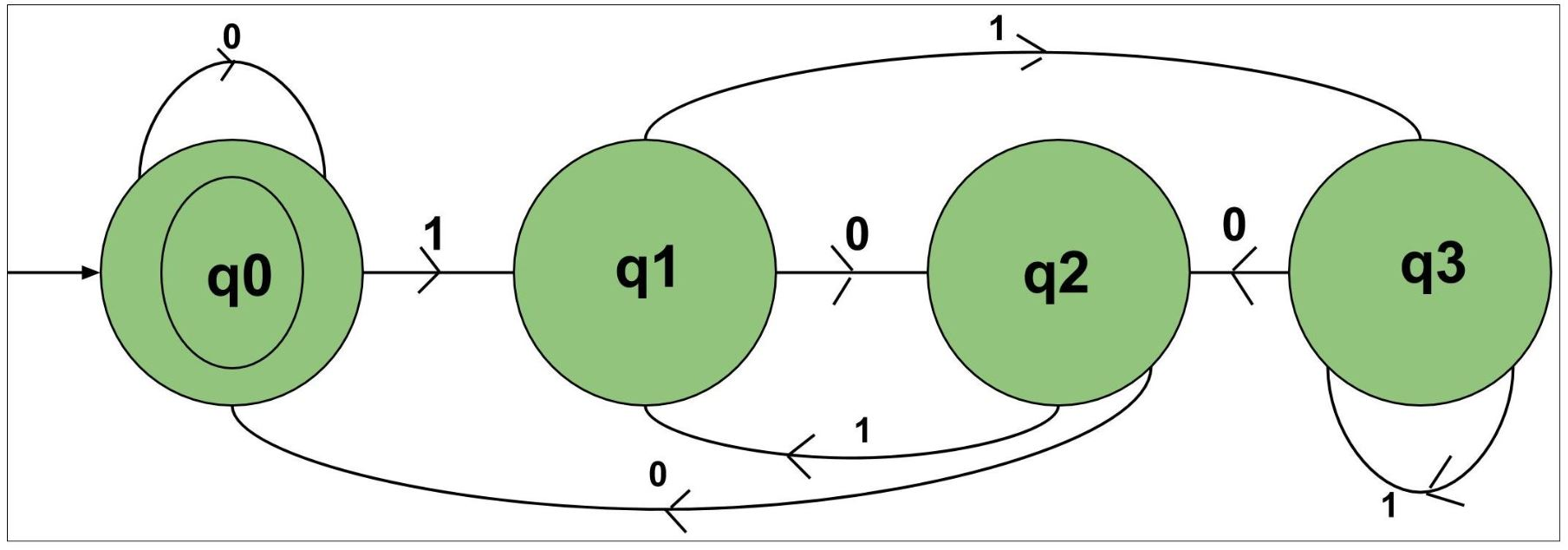Related Articles
Construct DFA which interpreted as binary number is divisible by 2, 3, 4
• Last Updated : 28 Jun, 2020

Prerequisite: Finite Automata Introduction, Designing Finite Automata
Problem-1:
Construct DFA, which accepts set of all strings over {0, 1} which interpreted as binary number is divisible by 2.

Explanation:
Consider the following inputs,

`{0, 01, 10, 11, 100, 101, 110........}`

The state transition diagram of the language will be like:In this DFA there are two states q0 and q1 and the input is strings of {0, 1} which is interpreted as binary number.
The state q0 is final state and q1 is non-final state. State q0 will be representing all the numbers that are divisible by 2, that is {0, 10, 100, 110…..}.
State q1 will be representing all the numbers that are not divisible by 2, that is {01, 11, 101, ……}.

 `def` `stateq0(n):``    ``#if length found 0 ``    ``#print not accepted``    ``if` `(``len``(n)``=``=``0``):``        ``print``(``"string accepted"``)``    ``else``:    ``         ` `        ``#if at index 0 ``        ``#'0' found call ``        ``#function stateq0 ``        ``if``(n[``0``]``=``=``'0'``):``            ``stateq0(n[``1``:])``             ` `        ``#else if '1' found ``        ``#call function q1.    ``        ``elif` `(n[``0``]``=``=``'1'``):``            ``stateq1(n[``1``:])`` ` `def` `stateq1(n):``    ``#if length found 0 ``    ``#print not accepted``    ``if` `(``len``(n)``=``=``0``):``        ``print``(``"string not accepted"``)``    ``else``:    ``         ` `        ``#if at index 0 ``        ``#'0' found call ``        ``#function stateq0 ``        ``if``(n[``0``]``=``=``'0'``):``            ``stateq0(n[``1``:])``             ` `        ``#else if '1' found ``        ``#call function q1.    ``        ``elif` `(n[``0``]``=``=``'1'``):``            ``stateq1(n[``1``:])            ``         ` ` ` `#take number from user``n``=``int``(``input``())``#converting number to binary``n ``=` `bin``(n).replace(``"0b"``, "") `` ` `#call stateA``#to check the input``stateq0(n)`
```INPUT: 5
OUTPUT: String Not Accepted
```

The above automata will accept set of all strings over {0, 1} which when interpreted as binary number is divisible by 2.
Whenever the number is not divisible by 2 then it will go from state q0 to q1. When the number is divisible by 2, then it will go from state q1 to q0 or if it was initially in q0 then it will accept it.

Problem-2:
Construct DFA, which accepts set of all strings over {0, 1} which interpreted as binary number is divisible by 3.

Explanation:
Refer for solution: Binary string multiple of 3 using DFA.

Problem-3:
Construct DFA, which accepts set of all strings over {0, 1} which interpreted as binary number is divisible by 4.

Explanation:
Consider the following inputs,

`{0, 01, 10, 11, 100, 101, 110........}`

The state transition diagram of the language will be like:Explanation:
In this DFA there are three states q0, q1, q2, q3 and the input is strings of {0, 1} which is interpreted as binary number. The state q0 is final state and q1, q2, q3 are non-final state.

• State q0 will be representing all the numbers that are divisible by 4, that is {0, 100, 1000…..}.
• State q1 will be representing all the numbers that are not divisible by 4 and give remainder 1 when divided by 4, that is {01, 101,,……}.
• State q2 will be representing all the numbers that are not divisible by 4 and give remainder 2 when divided by 4, that is {10, 110, ……}.
• State q4 will be representing all the numbers that are not divisible by 4 and give remainder 3 when divided by 4, that is {11, 111, ……}.

The above automata will accept set of all strings over {0, 1} which when interpreted as binary number is divisible by 4.

• State Whenever the number is not divisible by 4 and gives remainder of 1 then it will go to state q1.
• State Whenever the number is not divisible by 4 and gives remainder of 2 then it will go to state q2.
• State Whenever the number is not divisible by 4 and gives remainder of 3 then it will go to state q3.
• State Whenever the number is divisible by 4, then it will go to state q0 or if it was initially in q0 then it will accept it.
 `def` `stateq0(n):``    ``#if length found 0 ``    ``#print not accepted``    ``if` `(``len``(n)``=``=``0``):``        ``print``(``"string accepted"``)``    ``else``:    ``         ` `        ``#if at index 0 ``        ``#'0' found call ``        ``#function stateq0 ``        ``if``(n[``0``]``=``=``'0'``):``            ``stateq0(n[``1``:])``             ` `        ``#else if '1' found ``        ``#call function q1.    ``        ``elif` `(n[``0``]``=``=``'1'``):``            ``stateq1(n[``1``:])`` ` `def` `stateq1(n):``    ``#if length found 0 ``    ``#print not accepted``    ``if` `(``len``(n)``=``=``0``):``        ``print``(``"string not accepted"``)``    ``else``:    ``         ` `        ``#if at index 0 ``        ``#'0' found call ``        ``#function stateq2 ``        ``if``(n[``0``]``=``=``'0'``):``            ``stateq2(n[``1``:])``             ` `        ``#else if '1' found ``        ``#call function q3.    ``        ``elif` `(n[``0``]``=``=``'1'``):``            ``stateq3(n[``1``:])            ``         ` `def` `stateq2(n):``    ``#if length found 0 ``    ``#print not accepted``    ``if` `(``len``(n)``=``=``0``):``        ``print``(``"string not accepted"``)``    ``else``:    ``         ` `        ``#if at index 0 ``        ``#'0' found call ``        ``#function stateq0 ``        ``if``(n[``0``]``=``=``'0'``):``            ``stateq0(n[``1``:])``             ` `        ``#else if '1' found ``        ``#call function q1.    ``        ``elif` `(n[``0``]``=``=``'1'``):``            ``stateq1(n[``1``:])   ``             ` `def` `stateq3(n):``    ``#if length found 0 ``    ``#print not accepted``    ``if` `(``len``(n)``=``=``0``):``        ``print``(``"string not accepted"``)``    ``else``:    ``         ` `        ``#if at index 0 ``        ``#'0' found call ``        ``#function stateq2``        ``if``(n[``0``]``=``=``'0'``):``            ``stateq2(n[``1``:])``             ` `        ``#else if '1' found ``        ``#call function q3.    ``        ``elif` `(n[``0``]``=``=``'1'``):``            ``stateq3(n[``1``:])            ``                     ` `                 ` ` ` `#take number from user``n``=``int``(``input``())``#converting number to binary``n ``=` `bin``(n).replace(``"0b"``, "") `` ` `#call stateA``#to check the input``stateq0(n)`
My Personal Notes arrow_drop_up